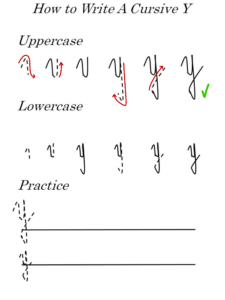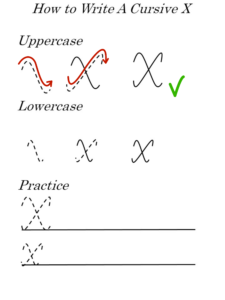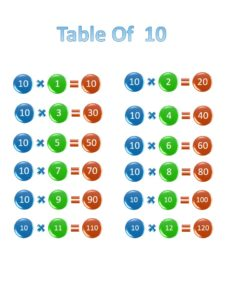## Printable 10 times table, chart, and practice worksheets for multiplication

The 10 times table is among the easiest ones to learn. Still, coming from the table of 9, this can be seen as one of the hardest ones. That’s...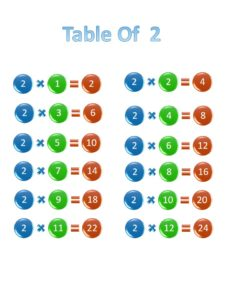## Printable 2 times table and practice worksheets for multiplication

Usually, the 2 times table is the first real multiplication table they learn. The table of 1 is easy, but it’s just used to explain the concept of multiplication....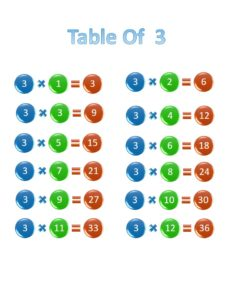## Printable 3 times table and practice worksheets for multiplication

Find here the best printable 3 times multiplication table, a great worksheet to practice, and awesome tips to learn them. These are the most useful to master the table...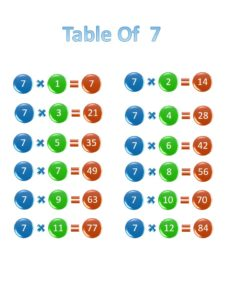## Printable 7 times table, chart, and practice worksheets for multiplication

The 7 times multiplication table is the first one among the tables that are usually perceived as ‘difficult to learn’ for kids. Don’t let your kids have a rough...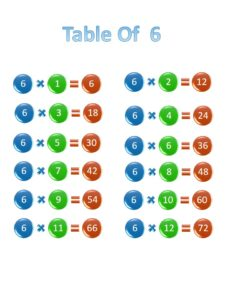## Printable 6 times table, chart, and practice worksheets for multiplication

Find here the best worksheets and charts for kids to learn the 6 times table easily at home. These are very useful to kids struggling with math at school....## Printable 12 times table, chart, and practice worksheets for multiplication

The 12 times table is usually the last multiplication table kids learn in school; thus, it’s seen as the most difficult to master. That’s why we’ve created the most...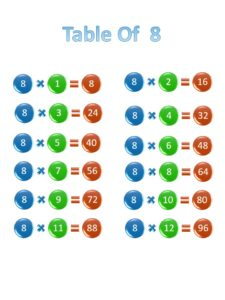## Printable 8 times table, chart, and practice worksheets for multiplication

Are you looking for some printable 8 times multiplication table and chart? Find here, not only that but also the best missing factor worksheets to help your kids learn...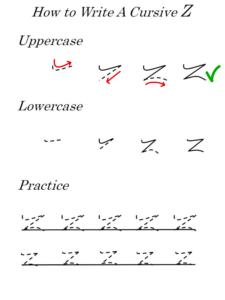## Cursive ‘Z’ practice worksheets: Learn to write the letter ‘Z’ in uppercase and lowercase

Are you looking for some letter ‘Z’ worksheets? This exercise sheet will prove to be efficient in the mastery of this simple-looking letter. We can assure you that your...